We use cookies to provide you with a great user experience. By using our site, you accept our use of cookies. You can review our cookie and privacy policy here.
• Support

Our Customer Service team is available from 8am to 6:00pm, ET, Monday through Friday. Live chat is available from 8am to 5:30pm ET, Monday-Friday.

Call:
800.334.5551
Fax:
Email:
Email Customer Service

• Cart
0

### My Cart

Your Shopping Cart is currently empty. Use Quick Order or Search to quickly add items to your order!

# Physical Science Math Review: Techniques, Formulae, and Constants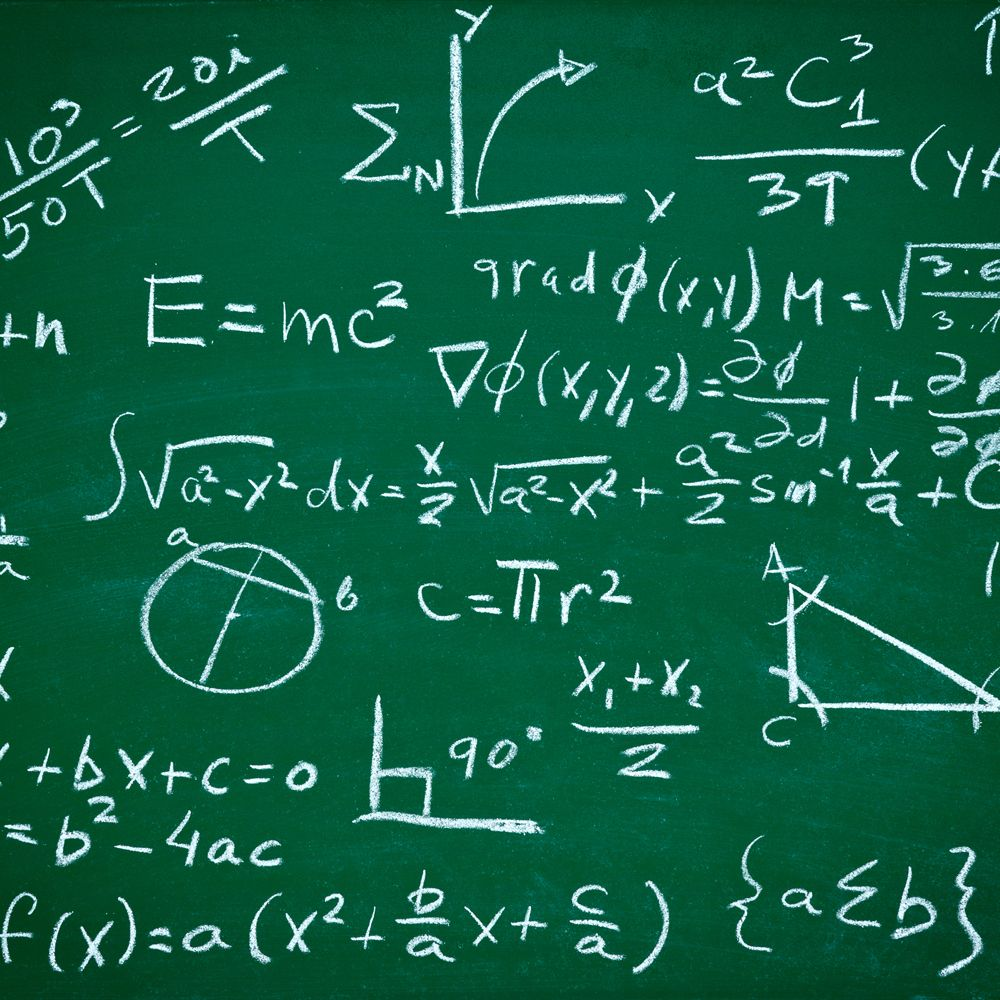Dee Dee Whitaker
Product Content Specialist

March 2017

Having good math skills is important and necessary for students to be successful in physical science courses. As a course progresses, formulae build up, units become more complex, and students may become confused. This Physical Science Math Review can help students organize formulae by topic and recall the appropriate SI units; it also gives them a metric conversion and scientific notation refresher. Use the math review from day one or as an end-of-course exam review. Math is the language of science.

### Metric scale and conversions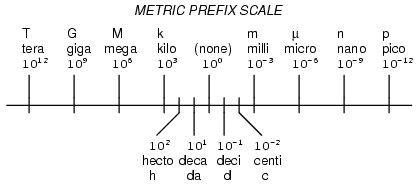Conversion factor method:
Given quantity × conversion factor = desired unit
Given quantity × desired unit = desired unit
given unit

Example:
Convert 450 mL to L.Metric prefix exponent method:
(Exponent of given unit) – (exponent of desired unit) = number of places and direction to move decimal point
Negative answer: move the decimal to the left

Example:
Convert 450 mL to L.
milli = –3    Liter = 0
(–3) – (0) = –3
Move the decimal point 3 places to the left from its original position: 0.450 L

### Scientific notation

Scientific notation converts a large or small number into a number with a mantissa between 1 and 10 multiplied by 10 raised to an exponent.

Steps:

1. Move the decimal point in the original number to create a new number between 1.0 and 9.99.
2. The number of places you moved the decimal point becomes the exponent. If you moved the decimal point to the right, the exponent is negative. If you moved the decimal point to the left, the exponent is positive.

Example:
Place 234,000 into scientific notation.
The decimal is behind the last 0 and must be moved 5 places to the left.
The answer is 2.34 × 105.

### Formulae and formula manipulation

Problem-solving steps:

1. List the given information and the UNITS.
2. List the unknown or variable you need to solve for.
3. Select the formula that contains the given units and the unknown.
4. Manipulate the formula to isolate the unknown.
5. Plug in the numbers with units.
6. Cross out like units (unit analysis). The unit for the unknown should remain.
7. Perform the necessary arithmetic.
8. Make sure the final answer has a number and a unit.

Example:
If a sample of a substance has a density of 2.1 g/mL and a mass of 8.0 g, what is its volume?
Given: D = 2.1 g/mL    m = 8.0 g
Unknown = volume mL
D = m/V   so   V = m/D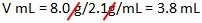### Motion, energy, and work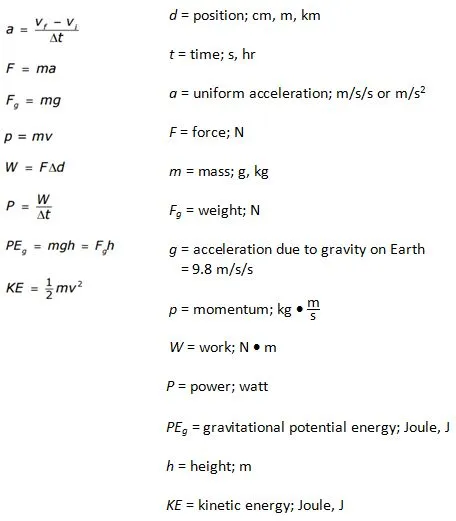### Machines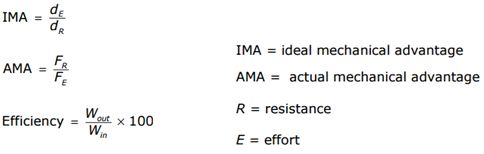### Waves and the electromagnetic spectrum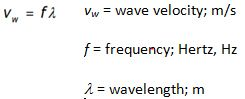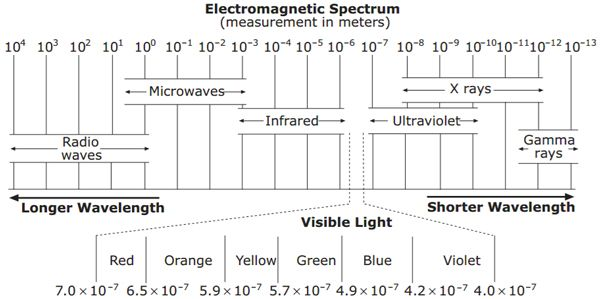###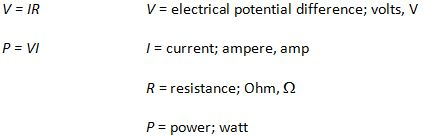Density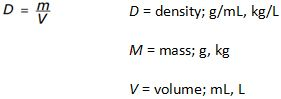### Temperature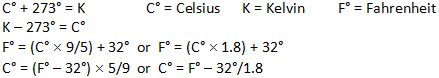### Gas laws

1. Boyle’s Law  P1V1 = P2V2                                              P = Pressure in atm, mmHg, or Pa, kPa
2. Charles’s Law  V1/T1  = V2/T2                                       V = Volume in mL or L
3. Combined Gas Law  (P1V1/T1) = (P2V2/T2)                T = Temperature and must be in Kelvin

### Thermodynamics

1. q = m × Cp × DT                                q = quantity of heat; Joule, J          m = mass in g or kg
2. q = m Hv                                             Cp = Specific heat in J/gC° or kJ/kgC° or cal/gC°
3. q = mHf                                               Hv = Heat of vaporization in J/g or kJ/kg
Hf = Heat of fusion in J/g or kJ/kg

Specific Heat in J/gC°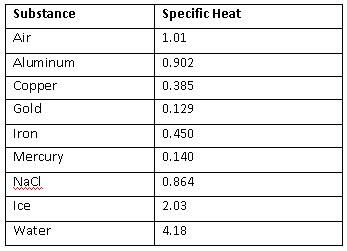Summary of Radiation Types and Properties

 Type of Radiation Alpha particle Beta particle Gamma ray Symbol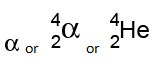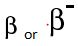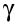Mass (atomic mass units) 4 1/2000 0 Charge +2 –1 0 Speed slow fast very fast (speed of light) Ionizing ability high medium 0 Penetrating power low medium high Stopped by paper aluminum lead

Balancing nuclear equations:

• All substances must be written with mass and nuclear charge.
• Mass must balance (top number).
• Charge must balance (bottom number).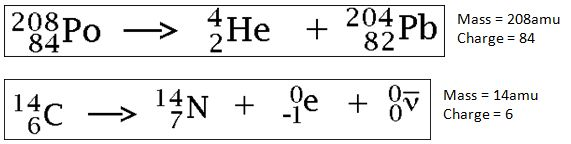### Major types of chemical reactions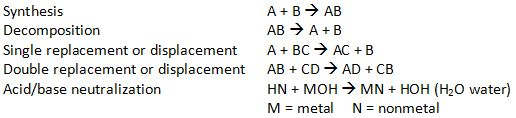### Mole conversions

1 mole = 6.02 × 1023 particles              particles may include atoms, molecules, ions, electrons
1 mole of a gas at Standard Temperature and Pressure (STP) occupies 22.4 L
Conversion factors: grams/molar mass = moles = particles/6.02 × 1023 particles

### Acids and bases

pH =  –log[H+]  or  pH =  –log[H3O+]                     [ ] = concentration
pOH =  –log[OH-]
pH + pOH =14
Kw = [H+] × [OH-] = 1 × 10-14  at 25°C